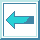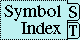### 22.2.2 Examples of using the Pretty Printer

As an example of the interaction of logical blocks, conditional newlines, and indentation, consider the function simple-pprint-defun below. This function prints out lists whose cars are defun in the standard way assuming that the list has exactly length 4.

```(defun simple-pprint-defun (*standard-output* list)
(pprint-logical-block (*standard-output* list :prefix "(" :suffix ")")
(write (first list))
(write-char #\Space)
(pprint-newline :miser)
(pprint-indent :current 0)
(write (second list))
(write-char #\Space)
(pprint-newline :fill)
(write (third list))
(pprint-indent :block 1)
(write-char #\Space)
(pprint-newline :linear)
(write (fourth list))))
```

Suppose that one evaluates the following:

```(simple-pprint-defun *standard-output* '(defun prod (x y) (* x y)))
```

If the line width available is greater than or equal to 26, then all of the output appears on one line. If the line width available is reduced to 25, a line break is inserted at the linear-style conditional newline before the expression (* x y), producing the output shown. The (pprint-indent :block 1) causes (* x y) to be printed at a relative indentation of 1 in the logical block.

``` (DEFUN PROD (X Y)
(* X Y))
```

If the line width available is 15, a line break is also inserted at the fill style conditional newline before the argument list. The call on (pprint-indent :current 0) causes the argument list to line up under the function name.

```(DEFUN PROD
(X Y)
(* X Y))
```

If *print-miser-width* were greater than or equal to 14, the example output above would have been as follows, because all indentation changes are ignored in miser mode and line breaks are inserted at miser-style conditional newlines.

``` (DEFUN
PROD
(X Y)
(* X Y))
```

As an example of a per-line prefix, consider that evaluating the following produces the output shown with a line width of 20 and *print-miser-width* of nil.

``` (pprint-logical-block (*standard-output* nil :per-line-prefix ";;; ")
(simple-pprint-defun *standard-output* '(defun prod (x y) (* x y))))

;;; (DEFUN PROD
;;;        (X Y)
;;;   (* X Y))
```

As a more complex (and realistic) example, consider the function pprint-let below. This specifies how to print a let form in the traditional style. It is more complex than the example above, because it has to deal with nested structure. Also, unlike the example above it contains complete code to readably print any possible list that begins with the symbol let. The outermost pprint-logical-block form handles the printing of the input list as a whole and specifies that parentheses should be printed in the output. The second pprint-logical-block form handles the list of binding pairs. Each pair in the list is itself printed by the innermost pprint-logical-block. (A loop form is used instead of merely decomposing the pair into two objects so that readable output will be produced no matter whether the list corresponding to the pair has one element, two elements, or (being malformed) has more than two elements.) A space and a fill-style conditional newline are placed after each pair except the last. The loop at the end of the topmost pprint-logical-block form prints out the forms in the body of the let form separated by spaces and linear-style conditional newlines.

``` (defun pprint-let (*standard-output* list)
(pprint-logical-block (nil list :prefix "(" :suffix ")")
(write (pprint-pop))
(pprint-exit-if-list-exhausted)
(write-char #\Space)
(pprint-logical-block (nil (pprint-pop) :prefix "(" :suffix ")")
(pprint-exit-if-list-exhausted)
(loop (pprint-logical-block (nil (pprint-pop) :prefix "(" :suffix ")")
(pprint-exit-if-list-exhausted)
(loop (write (pprint-pop))
(pprint-exit-if-list-exhausted)
(write-char #\Space)
(pprint-newline :linear)))
(pprint-exit-if-list-exhausted)
(write-char #\Space)
(pprint-newline :fill)))
(pprint-indent :block 1)
(loop (pprint-exit-if-list-exhausted)
(write-char #\Space)
(pprint-newline :linear)
(write (pprint-pop)))))
```

Suppose that one evaluates the following with *print-level* being 4, and *print-circle* being true.

``` (pprint-let *standard-output*
'#1=(let (x (*print-length* (f (g 3)))
(z . 2) (k (car y)))
(setq x (sqrt z)) #1#))
```

If the line length is greater than or equal to 77, the output produced appears on one line. However, if the line length is 76, line breaks are inserted at the linear-style conditional newlines separating the forms in the body and the output below is produced. Note that, the degenerate binding pair x is printed readably even though it fails to be a list; a depth abbreviation marker is printed in place of (g 3); the binding pair (z . 2) is printed readably even though it is not a proper list; and appropriate circularity markers are printed.

``` #1=(LET (X (*PRINT-LENGTH* (F #)) (Z . 2) (K (CAR Y)))
(SETQ X (SQRT Z))
#1#)
```

If the line length is reduced to 35, a line break is inserted at one of the fill-style conditional newlines separating the binding pairs.

``` #1=(LET (X (*PRINT-PRETTY* (F #))
(Z . 2) (K (CAR Y)))
(SETQ X (SQRT Z))
#1#)
```

Suppose that the line length is further reduced to 22 and *print-length* is set to 3. In this situation, line breaks are inserted after both the first and second binding pairs. In addition, the second binding pair is itself broken across two lines. Clause (b) of the description of fill-style conditional newlines (see the function pprint-newline) prevents the binding pair (z . 2) from being printed at the end of the third line. Note that the length abbreviation hides the circularity from view and therefore the printing of circularity markers disappears.

``` (LET (X
(*PRINT-LENGTH*
(F #))
(Z . 2) ...)
(SETQ X (SQRT Z))
...)
```

The next function prints a vector using ``#(...)'' notation.

```(defun pprint-vector (*standard-output* v)
(pprint-logical-block (nil nil :prefix "#(" :suffix ")")
(let ((end (length v)) (i 0))
(when (plusp end)
(loop (pprint-pop)
(write (aref v i))
(if (= (incf i) end) (return nil))
(write-char #\Space)
(pprint-newline :fill))))))
```

Evaluating the following with a line length of 15 produces the output shown.

``` (pprint-vector *standard-output* '#(12 34 567 8 9012 34 567 89 0 1 23))

#(12 34 567 8
9012 34 567
89 0 1 23)
```

As examples of the convenience of specifying pretty printing with format strings, consider that the functions simple-pprint-defun and pprint-let used as examples above can be compactly defined as follows. (The function pprint-vector cannot be defined using format because the data structure it traverses is not a list.)

```(defun simple-pprint-defun (*standard-output* list)
(format T "~:<~W ~@_~:I~W ~:_~W~1I ~_~W~:>" list))

(defun pprint-let (*standard-output* list)
(format T "~:<~W~^~:<~@{~:<~@{~W~^~_~}~:>~^~:_~}~:>~1I~@{~^~_~W~}~:>" list))
```

In the following example, the first form restores *print-pprint-dispatch* to the equivalent of its initial value. The next two forms then set up a special way to pretty print ratios. Note that the more specific type specifier has to be associated with a higher priority.

``` (setq *print-pprint-dispatch* (copy-pprint-dispatch nil))

(set-pprint-dispatch 'ratio
#'(lambda (s obj)
(format s "#.(/ ~W ~W)"
(numerator obj) (denominator obj))))

(set-pprint-dispatch '(and ratio (satisfies minusp))
#'(lambda (s obj)
(format s "#.(- (/ ~W ~W))"
(- (numerator obj)) (denominator obj)))
5)

(pprint '(1/3 -2/3))
(#.(/ 1 3) #.(- (/ 2 3)))
```

The following two forms illustrate the definition of pretty printing functions for types of code. The first form illustrates how to specify the traditional method for printing quoted objects using single-quote. Note the care taken to ensure that data lists that happen to begin with quote will be printed readably. The second form specifies that lists beginning with the symbol my-let should print the same way that lists beginning with let print when the initial pprint dispatch table is in effect.

``` (set-pprint-dispatch '(cons (member quote)) ()
#'(lambda (s list)
(if (and (consp (cdr list)) (null (cddr list)))
(funcall (formatter "'~W") s (cadr list))
(pprint-fill s list))))

(set-pprint-dispatch '(cons (member my-let))
(pprint-dispatch '(let) nil))
```

The next example specifies a default method for printing lists that do not correspond to function calls. Note that the functions pprint-linear, pprint-fill, and pprint-tabular are all defined with optional colon-p and at-sign-p arguments so that they can be used as pprint dispatch functions as well as ~/.../ functions.

``` (set-pprint-dispatch '(cons (not (and symbol (satisfies fboundp))))
#'pprint-fill -5)

;; Assume a line length of 9
(pprint '(0 b c d e f g h i j k))
(0 b c d
e f g h
i j k)
```

This final example shows how to define a pretty printing function for a user defined data structure.

``` (defstruct family mom kids)

(set-pprint-dispatch 'family
#'(lambda (s f)
(funcall (formatter "~@<#<~;~W and ~2I~_~/pprint-fill/~;>~:>")
s (family-mom f) (family-kids f))))
```

The pretty printing function for the structure family specifies how to adjust the layout of the output so that it can fit aesthetically into a variety of line widths. In addition, it obeys the printer control variables *print-level*, *print-length*, *print-lines*, *print-circle* and *print-escape*, and can tolerate several different kinds of malformity in the data structure. The output below shows what is printed out with a right margin of 25, *print-pretty* being true, *print-escape* being false, and a malformed kids list.

``` (write (list 'principal-family
(make-family :mom "Lucy"
:kids '("Mark" "Bob" . "Dan")))
:right-margin 25 :pretty T :escape nil :miser-width nil)
(PRINCIPAL-FAMILY
#<Lucy and
Mark Bob . Dan>)
```

Note that a pretty printing function for a structure is different from the structure's print-object method. While print-object methods are permanently associated with a structure, pretty printing functions are stored in pprint dispatch tables and can be rapidly changed to reflect different printing needs. If there is no pretty printing function for a structure in the current pprint dispatch table, its print-object method is used instead.

The following X3J13 cleanup issue, not part of the specification, applies to this section: